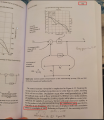# phase condition must be multiple of 360 to be stable in negative feedback?

#### TarikElec

Joined Oct 17, 2019
82
Hello everyone,
Reading this book about negative feedback loop, the Author mentioned that as in the red box that all the sum of phases( loop gain=commulative gain) must be multiple of 360 degrees which confuses me.
I know that when the feedback is at -180 phases and subtracting from the input signal makes the difference V(in+)-V(in-) increasing which leads to oscillations but here he is mentioning 360!Last edited:

#### Veracohr

Joined Jan 3, 2011
765
It’s saying that the phase isn’t the only factor that leads to oscillation. If the phase is a multiple of 360 but the gain is less than unity, oscillation won’t occur.

#### Papabravo

Joined Feb 24, 2006
18,793
If you take sine wave and shift it by 360° you have a replica of the original. If you subtract one from the other, you get zero. that is the definition of periodic. If you now sift that original sinewave by 180° and subtract them, there are occasional points where the answer is zero, but you also have points where the result is larger than either of the inputs.

#### AnalogKid

Joined Aug 1, 2013
10,096
the Author mentioned that as in the red box that all the sum of phases( loop gain=commulative gain) must be multiple of 360 degrees
No.

That is not at all what the author said. In fact, it is the opposite. There are a lot of words between your two red boxes, and those words are important. For example, the five words after the first box: must be less than unity

ak

#### Audioguru again

Joined Oct 21, 2019
4,793
I think the author is trying to say that when all the phase shifts in the loop total a multiple of 360 degrees then the amplifier gain must be less than unity which is like in all opamp circuits. The author forgot to say that since the feedback is negative then it already has 180 degrees of phase shift.

#### Papabravo

Joined Feb 24, 2006
18,793
It’s saying that the phase isn’t the only factor that leads to oscillation. If the phase is a multiple of 360 but the gain is less than unity, oscillation won’t occur.
Those are necessary conditions, but they may not be sufficient in all cases. Sometimes getting oscillators to start can be a challenge.

#### Ian0

Joined Aug 7, 2020
5,510
Another way of putting it:
if the gain is more than unity at any frequency where the phase shift is a multiple of 360°, then it is likely to oscillate (but probably not, if what you wanted it to do was oscillate)